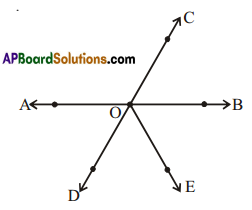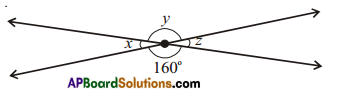AP State Syllabus AP Board 7th Class Maths Solutions Chapter 4 Lines and Angles Ex 6 Textbook Questions and Answers.

## AP State Syllabus 7th Class Maths Solutions 4th Lesson Lines and Angles Exercise 6Question 1.
Name two pairs of vertically opposite angles in the figure.Solution:
Vertically opposite angles are
∠AOC, ∠BOD and ∠BOC, ∠AODQuestion 2.
Find the measure of x, y and z without actually measuring them.Solution:
From the figure
∠y and 160° are vertically opposite angles and hence equal.
∴ ∠y=1600
Also ∠x = ∠z and (. vertically opposite angles)
x + 160° = 180° (Linear pair of angles)
∴ ∠x = 180° – 160° = 20°
∠z = 20° (∵ ∠x, ∠z are vertically opposite)
∴ x = 20°
y = 160°
z = 20°

Question 3.
Give some examples of vertically opposite angles in your surroundings.
Solution:
i) Angles between legs of a folding cot/scamp cot.
ii) Angles between legs of a folding chair.
iii) Angles between plates of a scissors.